# 4. One example.

Consider the equation form the last partWe expand y(x) in a perturbation seriesInsertion in the equation yields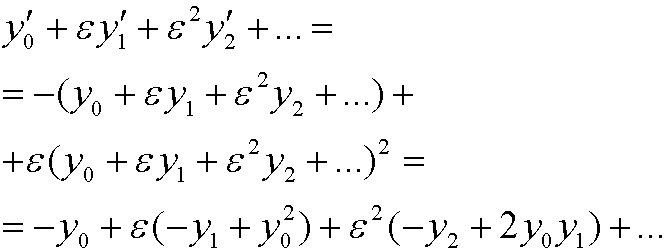Insertion in the inital condition yieldsNow letThenthat is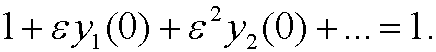If we subtract 1 on both sides and divide the rest with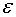we getOn more time, we letThen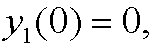and if we repeat the procedure we realize that also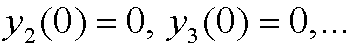We determine y0, y1, y2,…. subsequently by solving the equations that we get by comparing terms containing the same power of: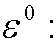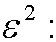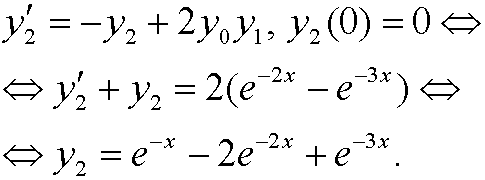An approximate solution is thus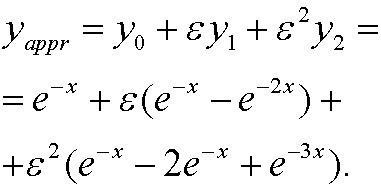www.larserikpersson.se